# 你能抓住这只猫吗?--HarmonyOS纯ets语言实现抓住神经猫游戏 原创精华

5收藏

## 1.前言

<<抓住神经猫>>游戏相信不少朋友都玩过,最近不是ARKUI挺火热的么,一直搞嵌入式的也想尝尝鲜,想找个小玩意入门,想起来5,6年前看过的一篇文章,分析神经猫的寻路算法,号称是最难抓住的神经猫,回去一看,这项目不正好练手吗,说干就干.

## 2.效果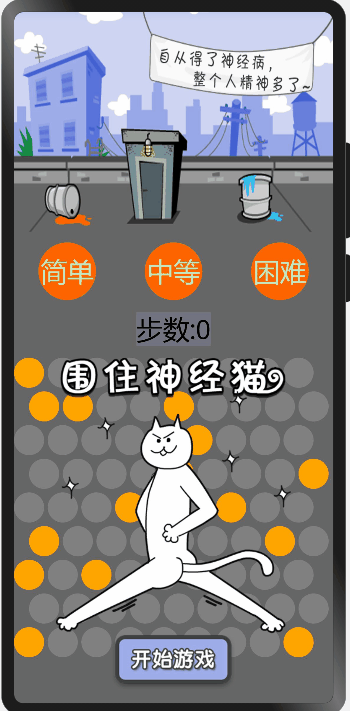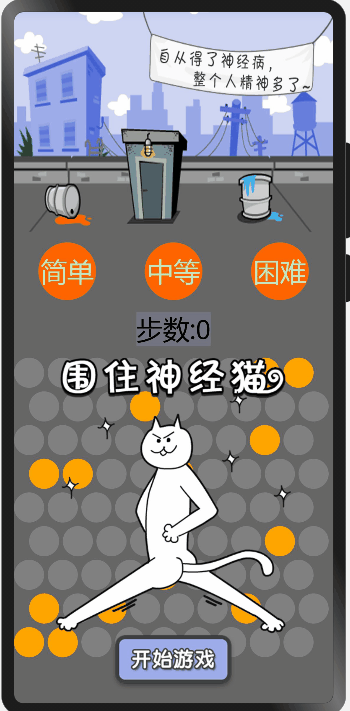## 3.关键部分解读

### 3.3 部分代码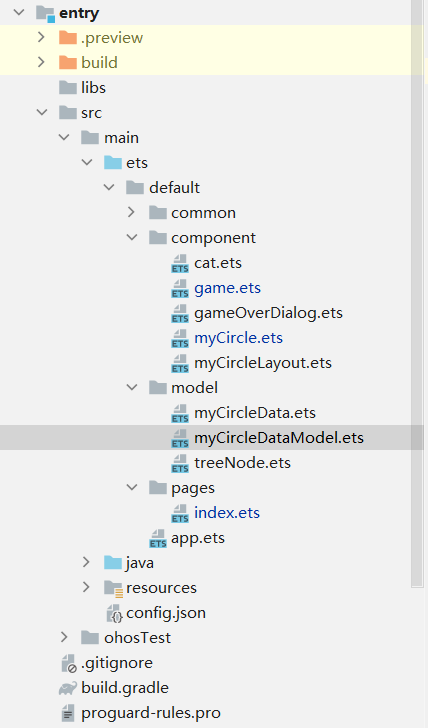``````
@Observed
export class catData{
pos_x:number
pos_y:number

location:number
step:number = 0

inWall:boolean=false

constructor(loc:number = defaultLocation){
this.setLocation(loc)
}

reset(){
this.inWall = false
this.step = 0
this.setLocation(defaultLocation)
}

setLocation(loc:number){
console.log("set Loction:"+loc)
this.location=loc
this.setPosition()
}

setPosition(){
let col:number = Math.floor(this.location/9)
let row:number = this.location%9

this.pos_x = (col%2 ? row*38+17:row*38)
this.pos_y = col*38

//    console.log("x:"+this.pos_x+" y:"+this.pos_y)
}

moveTo(location:number) {

this.setLocation(location)
this.step++

}

catInWall(yes:boolean=true){
this.inWall = yes
}
}

``````

### 3.3 主要逻辑

``````
export function onItemClick(index : number){
console.log("onItemClick "+index)
if(myCircleDataArray[index].clicked ){
console.log("不能点击")
return;

}
myCircleDataArray[index].setClick()

let catLocation = myCat.location

myCircleDataArray.forEach(item=>{
item.path = -100
item.depth = 100
})

myCircleDataArray[catLocation].depth=0

let neighbors = getNeighbors(catLocation,myCircleDataArray[catLocation].depth)

neighbors.forEach(item=> {

myCircleDataArray[item.location].depth=item.depth

})

let neighborList:Array<Neighbor> =neighbors

while(neighborList.length > 0){
let neighborsArrayList:Array<Neighbor> = []

neighborList.forEach(item=>{
let neighborsTemp = buildNeighborChild(item)
neighborsTemp.forEach(item=>{
neighborsArrayList.push(item)
})
})

neighborList = neighborsArrayList

}
neighbors.forEach(item=>{

calcCirclePath(item)

})

let best = getBestNeighbor(neighbors)

console.log("the best neighbor is:"+best.location+" path="+best.path);
if(best.path == 100){
console.log("cat is in wall");
myCat.catInWall()
myGame.setState(gameState.gameVictory)
}

myCat.moveTo(best.location)

if(catCheckLocation() == true){
console.log("cat escaped")
myGame.setState(gameState.gameFailed)
}

neighbors.length = 0

}
``````

``````function getBestNeighbor(neighbors:Neighbor[]):Neighbor{

let neighbor:Neighbor = neighbors

for(var i=1;i < neighbors.length;i++){
if(neighbor.path > neighbors[i].path){
neighbor = neighbors[i]
}
}
console.log("the shortest nei:"+neighbor.location)

//select the child more than other
for(var j=0;j < neighbors.length;j++){
if(neighbor.path == neighbors[j].path){
console.log("find same path:"+neighbors[j].location)
if(neighbor.child.length < neighbors[j].child.length){

neighbor = neighbors[j]
console.log("changed neighbor:"+neighbor.location)
}
}
}

return neighbor
}
``````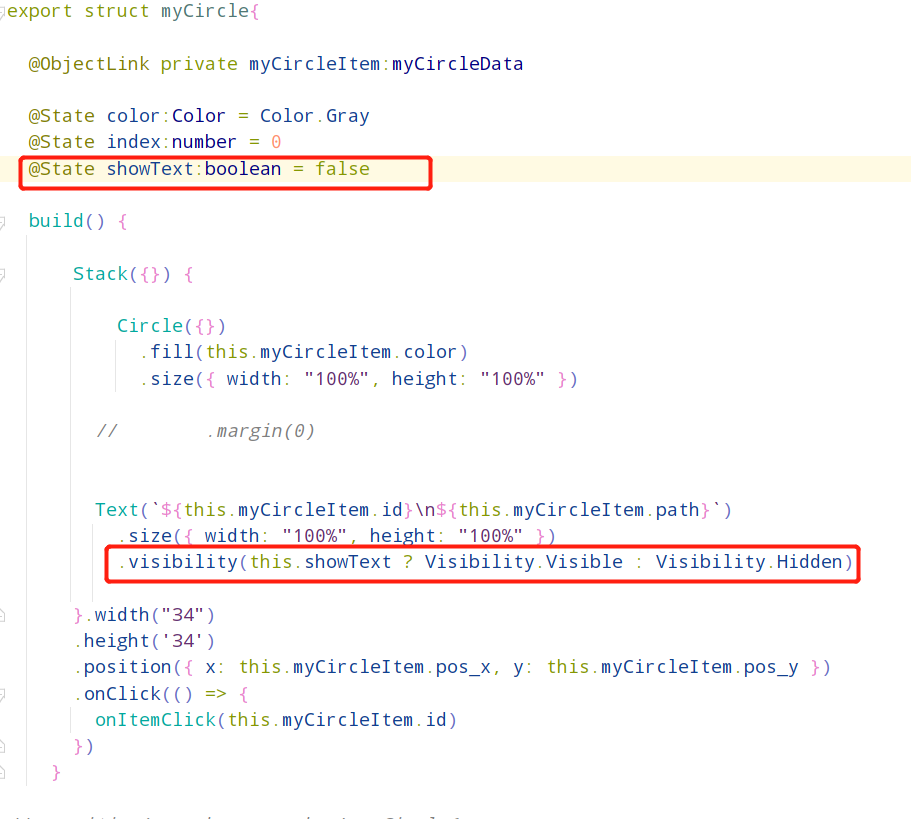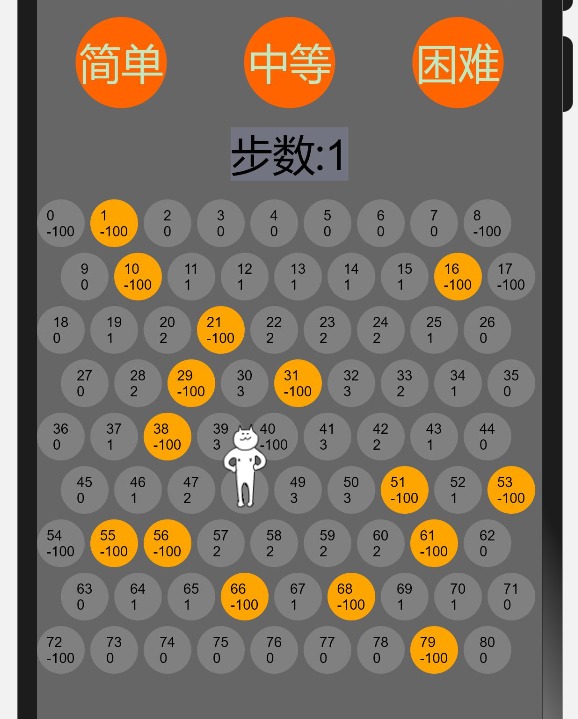## 4.补充

### 4.1 后续

1. 当前可以看到3个难度图标,目前还没做功能,后续会加上,难度的调节目前认为有几个地方:
初始墙的数量,逃跑算法的强度,或者优化初始墙的位置(简单的话就相对集中,困难的话就相对分散),以我非数学专业的功力感觉第一种靠谱一些,如果有数学大神也可以探讨一下后面两种怎么做
2. 可以看到猫逃跑的时候,从当前位置移动到下一位置,会出现短暂的消失,这有可能是模拟器在渲染动图的时候有什么延时导致的,后期准备研究一下动画效果,让猫的走动更顺滑
3. 由于目前还没有OpenHarmony能玩起来的开发板,所以只能是模拟器演示,后续如果有真正的开发板的话,相信很快看就能移植上去

### 4.2 关于代码

4.3 鸣谢

9

5条回复

/

2022-4-6 10:50:21

2022-4-6 20:17:16

2022-4-6 20:39:57
SummerRic

2022-4-7 14:05:15帖子
视频
声望
粉丝
热门推荐
社区精华内容